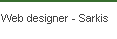Cornell University College Of Engineering
Email: aq23@cornell.edu

The Simulation

In the simulation of the pyloric circuit, Izhikevich's model of neural networks was employed, recognizing that his simulation is simply an approximation of the neuronal network Izhikevich's model can be better understood at his webpage (see works consulted). Below is a summary of the parameters used in Izhikevich's model: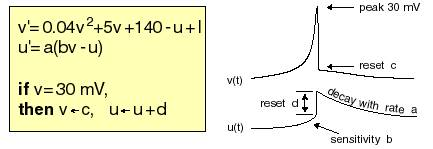In this model, the variable v represents the membrane voltage of the neuron, whilst the variable u is the membrane recovery variable, which accounts for the activation of K+ channels and inactivation of Na+ channels. The variable a shows the decay rate, b shows the sensitivity to spike, and c represents the reset voltage. In order to be able to tailor each of the six different categories of cells, the cell types were changes slightly, and the parameters of the cells were also changed in such a way as to produce the desired pattern (see diagram in Pyloric Circuit Link).

The parameters for the cells were changed as shown below. Note that the parameters on the right are from the original simulation whilst the parameters on the left are the parameters used for the simulation of the pyloric circuit.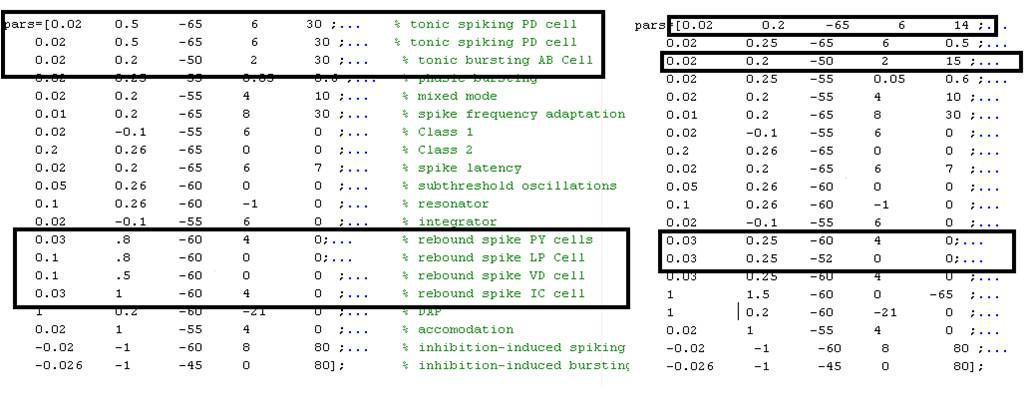Izhikevich's model was useful because it had already implemented the simulation of chemical synapses. I simply added the necessary cells to the SynStrength and SenseStrength matrices. Since there was no sensory input, the SenseStrength matrix was trivial. The SynStrength matrix was filled out as follows (based upon the circuit diagram shown under the Pyloric Circuit link):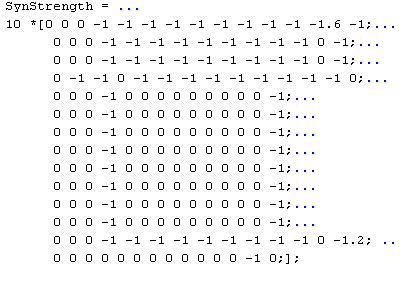Note the cells are in the following order: AB, PD, LP, PY, VD, and IC.

Izhikevich's model had one downside -- it did not account for electrical synapses. The Pyloric circuit consists of many electrical synapses, and this had to be accounted for in the simulation. Since Ohm's law states that V=IR, then we simply found Itrans = (V1-V2) * k ,where k is the conductance. The voltage was then updated by simply adding or subtracting the appropriate amount of current from the voltage updating step: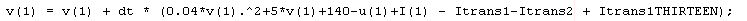The simulation then produced the following voltages (shown below). The actual recorded voltages are shown beside for comparison: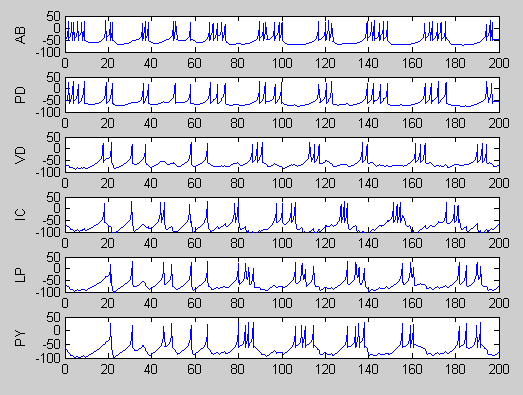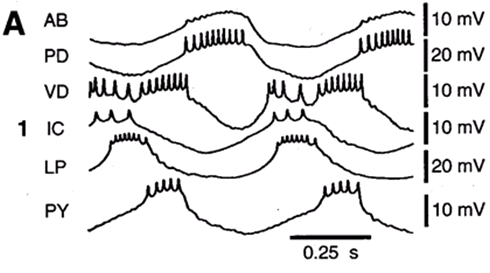With Dopamine in the Circuit, the empirical evidence produced the following results (according to experiments by Professor Harris-Warrick et al.):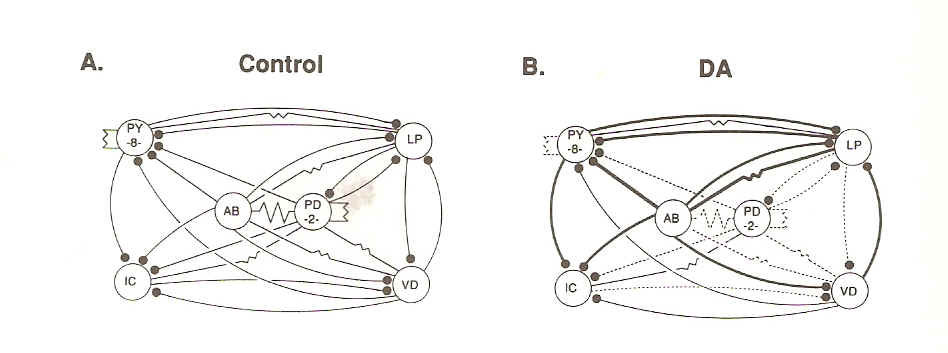According to "Distributed Effects of Dopamine Modulation in the Crustacean Pyloric Network" by Harris-Warrick et al. the following results should be obtained by dopamine in the STG: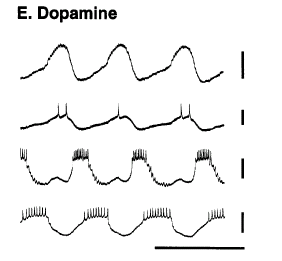Changing the synaptic strengths accordingly, the following graph was produced: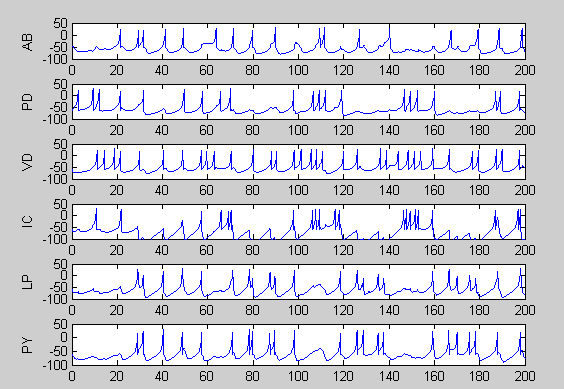Notice that even with much tweaking the desired effect is not remarkably obvious. The greatest error likely is due to the Izhikevich model itself -- since the model does not lend itself to adjusting the internal currents individually. The model simply lumps together the currents into to variables-- a and b -- making the effect of dopamine very difficult to model with this simulator.

In order to open the files right click and select "Save Link As", then open the simulation with Matlab. For the source code for the final simulation without dopamine click the link below:

Simulation 1

For the source code for the final simulation with dopamine click the link below:

 Web site design is proudly powered by FreelanceWebMarket.com© 2008 your company